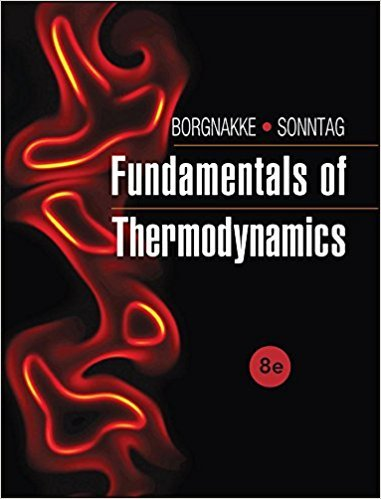×
Get Full Access to Fundamentals Of Thermodynamcs - 8 Edition - Chapter 3 - Problem 57hp
Get Full Access to Fundamentals Of Thermodynamcs - 8 Edition - Chapter 3 - Problem 57hp

×

# Consider a piston/cylinder setup with 0.5 kg of R-134a asISBN: 9781118131992 58

## Solution for problem 57HP Chapter 3

Fundamentals of Thermodynamcs | 8th Edition

• Textbook Solutions
• 2901 Step-by-step solutions solved by professors and subject experts
• Get 24/7 help from StudySoup virtual teaching assistantsFundamentals of Thermodynamcs | 8th Edition

4 5 1 339 Reviews
17
1
Problem 57HP

Problem 57HP

Consider a piston/cylinder setup with 0.5 kg of R-134a as saturated vapor at−10°C. It is now compressed to a pressure of 500 kPa in a polytropic process with n = 1.5. Find the final volume and temperature and determine the work done during the process.

Step-by-Step Solution:

Solution 57HP

Step 1 of 4

In this problem, we have to find the final volume, temperature and also determine the work done. The given data areis the pressureis the temperature and in kelvin it isis the mass wherepolytropic index.

Step 2 of 4

Step 3 of 4

##### ISBN: 9781118131992

Unlock Textbook Solution